Next: Monte Carlo simulation: MCB Up: PRODUCTION OF LINEARLY POLARISED Previous: Kinematics and cross sections

# Treatment of experimental deficiencies

Up to now an ideal electron beam was assumed, but in experiments a deficient electron beam (tab. 1) affects the photon spectra, especially the collimated ones. A finite beam spot size, characterised by the distribution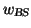of the impact positions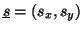of the electrons on the radiator, has the same effect like a collimator with a fuzzy edge and smears out the collimator cutoff in the photon spectra at xc (eq. 3b). The primary divergence of the electron beam, described by the distribution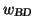, has a similar effect on xc but causes in addition a variation of the crystal angles with respect to their nominal values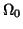changing the intensity due to the dependence of the momentum transfer on these angles. The deflection of the electron is not given by the beam divergence alone but is enhanced because the electron undergoes many small angle scattering processes mainly due to Coulomb interaction with atoms while traversing the radiator (thickness zR). This distribution is well represented by Molières theory, which uses a Gaussian approximation for small angles defined by the variance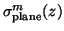being a function of medium properties and pathlength z, the particle has travelled.

 source distr. effect influence diamond temperature - Debye Waller factor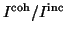BS: beam spot size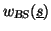"fuzzy" collimator xc BD: beam divergence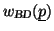+ variation ofxd MS: multiple scattering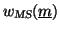increases BD xd ES: beam energy spread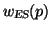smears out peaks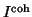The experimental photon intensity is a sum over all these effects weighted with the appropriate distributions. Due to the collimation condition (a collimator with radius rc is situated at distance zc) the boundary of the integration volume in eq. 4 is topological non-trivial.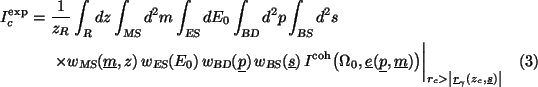Underlined vectors denote the transversal component of the respective unit vectors. This situation is sketched in fig. 2 in order to clarify the relations used to calculate the experimental spectra via eq. 4.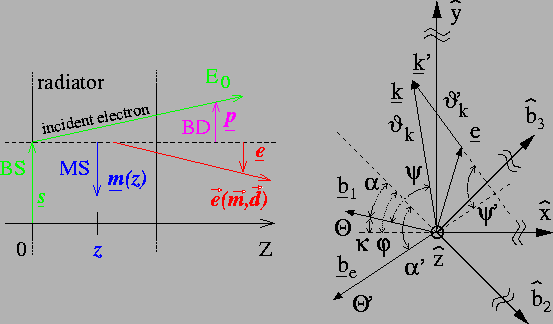Two approaches will be presented: (ii) An accurate Monte Carlo method: MCB which permits the study of collimation effects on the photon beam and its polarisation in full dependence of all electron beam deficiencies. (i) An analytical one: ANB which is approximative but very fast for quick first results.Next: Monte Carlo simulation: MCB Up: PRODUCTION OF LINEARLY POLARISED Previous: Kinematics and cross sections
Frank Natter
1999-07-16